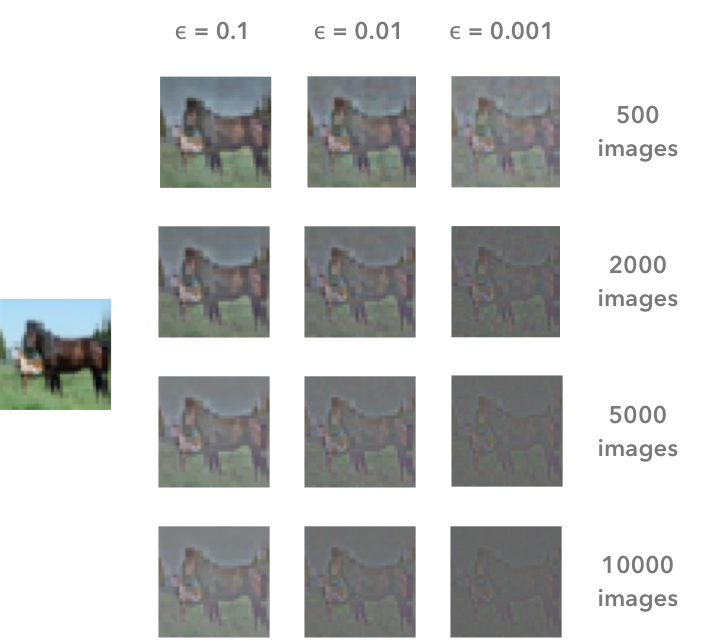1.背景：在第一部分中，我们将得到关于方差和协方差的一些提醒。我们将看到如何生成和绘制数据以更好地理解这些概念。

2.预处理：在第二部分中，我们将看到一些可应用于任何类型数据的预处理技术的基础知识 -  均值归一化标准化白化

3.白化图像：在第三部分中，我们将使用在1.2.中获得的工具和概念来进行称为零分量分析（ZCA）的特殊白化。它可用于预处理图像以进行深度学习。这部分将非常实用和有趣☃️！

## A.方差和协方差

n是矢量的长度，是向量的平均值。例如，A的第一列向量的方差是：

1. 和符号（Σ）意味着我们将迭代向量的元素。我们将从第一个元素（i = 1）开始，计算X的第一个元素减去向量X的平均值。

2.将结果乘以Y的第一个元素减去向量Y的平均值。

3.重复向量的每个元素的过程并计算所有结果的总和。

4.除以向量中的元素数量。

= 3ȳ= 4n = 3，所以我们有：

A = np.array([[1, 3, 5], [5, 4, 1], [3, 8, 6]])
A
array([[1, 3, 5],
[5, 4, 1],
[3, 8, 6]])

np.cov(A, rowvar=False, bias=True)
array([[ 2.66666667, 0.66666667, -2.66666667],
[ 0.66666667, 4.66666667, 2.33333333],
[-2.66666667, 2.33333333, 4.66666667]])

def calculateCovariance(X):
meanX = np.mean(X, axis = 0)
lenX = X.shape
X = X - meanX
covariance = X.T.dot(X)/lenX
return covariance

calculateCovariance(A)
array([[ 2.66666667, 0.66666667, -2.66666667],
[ 0.66666667, 4.66666667, 2.33333333],
[-2.66666667, 2.33333333, 4.66666667]])

## B.可视化数据和协方差矩阵

def plotDataAndCov(data):
ACov = np.cov(data, rowvar=False, bias=True)
print 'Covariance matrix:\n', ACov

fig, ax = plt.subplots(nrows=1, ncols=2)
fig.set_size_inches(10, 10)

ax0 = plt.subplot(2, 2, 1)

# Choosing the colors
cmap = sns.color_palette("GnBu", 10)
sns.heatmap(ACov, cmap=cmap, vmin=0)

ax1 = plt.subplot(2, 2, 2)

# data can include the colors
if data.shape==3:
c=data[:,2]
else:
c="#0A98BE"
ax1.scatter(data[:,0], data[:,1], c=c, s=40)

# Remove the top and right axes from the data plot
ax1.spines['right'].set_visible(False)
ax1.spines['top'].set_visible(False)


## C.模拟数据

np.random.seed(1234)
a1 = np.random.normal(2, 1, 300)
a2 = np.random.normal(1, 1, 300)
A = np.array([a1, a2]).T
A.shape
（300,2）

A[:10,:]
array([[ 2.47143516, 1.52704645],
[ 0.80902431, 1.7111124 ],
[ 3.43270697, 0.78245452],
[ 1.6873481 , 3.63779121],
[ 1.27941127, -0.74213763],
[ 2.88716294, 0.90556519],
[ 2.85958841, 2.43118375],
[ 1.3634765 , 1.59275845],
[ 2.01569637, 1.1702969 ],
[-0.24268495, -0.75170595]])

sns.distplot(A[:,0], color="#53BB04")
sns.distplot(A[:,1], color="#0A98BE")
plt.show()
plt.close()

plotDataAndCov(A)
plt.show()
plt.close()
Covariance matrix:
[[ 0.95171641 -0.0447816 ]
[-0.0447816 0.87959853]]

np.random.seed(1234)
b1 = np.random.normal(3, 1, 300)
b2 = b1 + np.random.normal(7, 1, 300)/2.
B = np.array([b1, b2]).T plotDataAndCov(B)
plt.show()
plt.close()
Covariance matrix:
[[ 0.95171641 0.92932561]
[ 0.92932561 1.12683445]]

## A.平均归一化

def center(X):
newX = X - np.mean(X, axis = 0)
return newX

BCentered = center(B)

print 'Before:\n\n'

plotDataAndCov(B)
plt.show()
plt.close()

print 'After:\n\n'

plotDataAndCov(BCentered)
plt.show()
plt.close()

Before:

Covariance matrix:
[[ 0.95171641 0.92932561]
[ 0.92932561 1.12683445]]

After:

Covariance matrix:
[[ 0.95171641  0.92932561]
[ 0.92932561  1.12683445]]


## B.标准化或归一化

def standardize(X):
newX = center(X)/np.std(X, axis = 0)
return newX

np.random.seed(1234)
c1 = np.random.normal(3, 1, 300)
c2 = c1 + np.random.normal(7, 5, 300)/2.
C = np.array([c1, c2]).T
plotDataAndCov(C) plt.xlim(0, 15)
plt.ylim(0, 15)
plt.show()
plt.close()
Covariance matrix:
[[ 0.95171641 0.83976242]
[ 0.83976242 6.22529922]]

CStandardized = standardize(C)
plotDataAndCov(CStandardized)
plt.show()
plt.close()
Covariance matrix:
[[ 1. 0.34500274]
[ 0.34500274 1. ]]

## C.白化

• 零中心数据
• 数据解相关
• 重新缩放数据

CCentered = center(C)
plotDataAndCov(CCentered)
plt.show()
plt.close()
Covariance matrix:
[[ 0.95171641 0.83976242]
[ 0.83976242 6.22529922]]

2.去相关

• 计算协方差矩阵
• 计算协方差矩阵的特征向量
• 将特征向量矩阵应用于数据 - 这将应用旋转

让我们将其打包到函数中：
def decorrelate(X):
newX = center(X)
cov = X.T.dot(X)/float(X.shape)
# Calculate the eigenvalues and eigenvectors of the covariance matrix
eigVals, eigVecs = np.linalg.eig(cov)
# Apply the eigenvectors to X
decorrelated = X.dot(eigVecs)
return decorrelated


plotDataAndCov(C)
plt.show()
plt.close()

CDecorrelated = decorrelate(CCentered)
plotDataAndCov(CDecorrelated)
plt.xlim(-5,5)
plt.ylim(-5,5)
plt.show()
plt.close()
Covariance matrix:
[[ 0.95171641 0.83976242]
[ 0.83976242 6.22529922]]
[[242  6.22529922]]


Covariance matrix:
[[  5.96126981e-01  -1.48029737e-16]
[ -1.48029737e-16   3.15205774e+00]]


3.重新缩放数据

def whiten(X):
newX = center(X)
cov = X.T.dot(X)/float(X.shape)
# Calculate the eigenvalues and eigenvectors of the covariance matrix
eigVals, eigVecs = np.linalg.eig(cov)
# Apply the eigenvectors to X
decorrelated = X.dot(eigVecs)
# Rescale the decorrelated data
whitened = decorrelated / np.sqrt(eigVals + 1e-5)
return whitened

CWhitened = whiten(CCentered)
plotDataAndCov(CWhitened)
plt.xlim(-5,5)
plt.ylim(-5,5)
plt.show()
plt.close()

Covariance matrix:
[[ 9.99983225e-01 -1.06581410e-16]
[ -1.06581410e-16 9.99996827e-01]]

CWhitened = whiten(CCentered)

plotDataAndCov(CWhitened)
plt.xlim(-5,5)
plt.ylim(-5,5)
plt.show()
plt.close()
Covariance matrix:
[[ 9.99983225e-01 -1.06581410e-16]
[ -1.06581410e-16 9.99996827e-01]]

## 3.图像白化

from keras.datasets import cifar10

(X_train, y_train), (X_test, y_test) = cifar10.load_data()

X_train.shape
(50000, 32, 32, 3)

CIFAR10数据集的训练集包含50000个图像。形状X_train(50000, 32, 32, 3)。每幅图像为32像素×32像素，每个像素包含3个维度（R，G，B）。每个值是0到255之间相应颜色的亮度。我们将从仅选择图像的一个子集开始，假设为1000：

X = X_train[:1000]
print X.shape
(1000, 32, 32, 3)

X = X.reshape(X.shape, X.shape*X.shape*X.shape)
print X.shape
(1000, 3072)

def plotImage(X):
plt.figure(figsize=(1.5, 1.5))
plt.imshow(X.reshape(32,32,3))
plt.show()
plt.close()

plotImage(X[12, :])

1.第一步是通过除以255（像素的最大值）来重新缩放图像以获得范围[0,1]。

X_norm = X / 255.
print 'X.min()', X_norm.min()
print 'X.max()', X_norm.max()

X.min() 0.0
X.max() 1.0

1.从所有图像中减去均值。

X_norm.mean(axis=0).shape
(3072,)

X_norm.mean(axis=0)
array([ 0.5234 , 0.54323137, 0.5274 , ..., 0.50369804,
0.50011765, 0.45227451])

 X_norm = X_norm - X_norm.mean(axis=0)

X_norm.mean(axis=0)
array([ -5.30575583e-16, -5.98021632e-16, -4.23439062e-16, ...,
-1.81965554e-16, -2.49800181e-16, 3.98570066e-17])

cov = np.cov(X_norm, rowvar=True)

U,S,V = np.linalg.svd(cov)

X是标准化的数据集，U是左奇异向量和S是图像归一化后的数据集的协方差的奇异值。ε是称为白化系数的超参数。diag（a）对应于一个矩阵，其中对角线为向量a，所有其他单元格为0。

print U.shape, S.shape
(1000, 1000) (1000,)

S是包含1000个元素的向量（奇异值）。因此，diag（S）的形状是(1000, 1000)，它的对角线为S

print np.diag(S)
print '\nshape:', np.diag(S).shape
[[ 8.15846654e+00 0.00000000e+00 0.00000000e+00 ..., 0.00000000e+00
0.00000000e+00 0.00000000e+00]
[ 0.00000000e+00 4.68234845e+00 0.00000000e+00 ..., 0.00000000e+00
0.00000000e+00 0.00000000e+00]
[ 0.00000000e+00 0.00000000e+00 2.41075267e+00 ..., 0.00000000e+00
0.00000000e+00 0.00000000e+00]
...,
[ 0.00000000e+00 0.00000000e+00 0.00000000e+00 ..., 3.92727365e-05
0.00000000e+00 0.00000000e+00]
[ 0.00000000e+00 0.00000000e+00 0.00000000e+00 ..., 0.00000000e+00
3.52614473e-05 0.00000000e+00]
[ 0.00000000e+00 0.00000000e+00 0.00000000e+00 ..., 0.00000000e+00
0.00000000e+00 1.35907202e-15]]

shape: (1000, 1000)

epsilon = 0.1
X_ZCA = U.dot(np.diag(1.0/np.sqrt(S + epsilon))).dot(U.T).dot(X_norm)
plotImage(X[12, :])
plotImage(X_ZCA[12, :])

X_ZCA_rescaled = (X_ZCA - X_ZCA.min()) / (X_ZCA.max() - X_ZCA.min())
print 'min:', X_ZCA_rescaled.min()
print 'max:', X_ZCA_rescaled.max()
min: 0.0
max: 1.0
plotImage(X[12, :])
plotImage(X_ZCA_rescaled[12, :])

## 参考资料

K. Jarrett, K. Kavukcuoglu, M. Ranzato, and Y. LeCun, “What is the best multi-stage architecture for object recognition?,” in 2009 IEEE 12th International Conference on Computer Vision, 2009, pp. 2146–2153.

A. Krizhevsky, “Learning Multiple Layers of Features from Tiny Images,” Master’s thesis, University of Tront, 2009.

Y. A. LeCun, L. Bottou, G. B. Orr, and K.-R. Müller, “Efficient BackProp,” in Neural Networks: Tricks of the Trade, Springer, Berlin, Heidelberg, 2012, pp. 9–48.

K. K. Pal and K. S. Sudeep, “Preprocessing for image classification by convolutional neural networks,” in 2016 IEEE International Conference on Recent Trends in Electronics, Information Communication Technology (RTEICT), 2016, pp. 1778–1781.

L. Wan, M. Zeiler, S. Zhang, Y. L. Cun, and R. Fergus, “Regularization of Neural Networks using DropConnect,” in International Conference on Machine Learning, 2013, pp. 1058–1066.

And also these great resources and QA:

Wikipedia - Whitening transformation

CS231 - Convolutional Neural Networks for Visual Recognition

Dustin Stansbury - The Clever Machine

Some details about the covariance matrix

Is this the correct way of whitening an image in python?

Mean normalization per image or from the entire dataset

Mean subtraction - all images or per image?

Why centering is important - See section 4.3

Kaggle kernel on ZCA

How ZCA is implemented in Keras